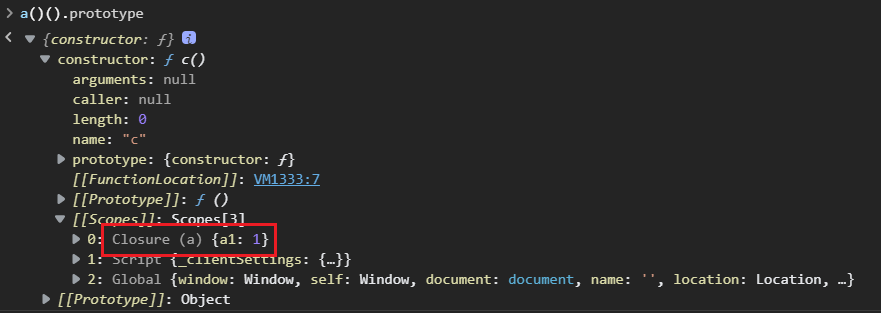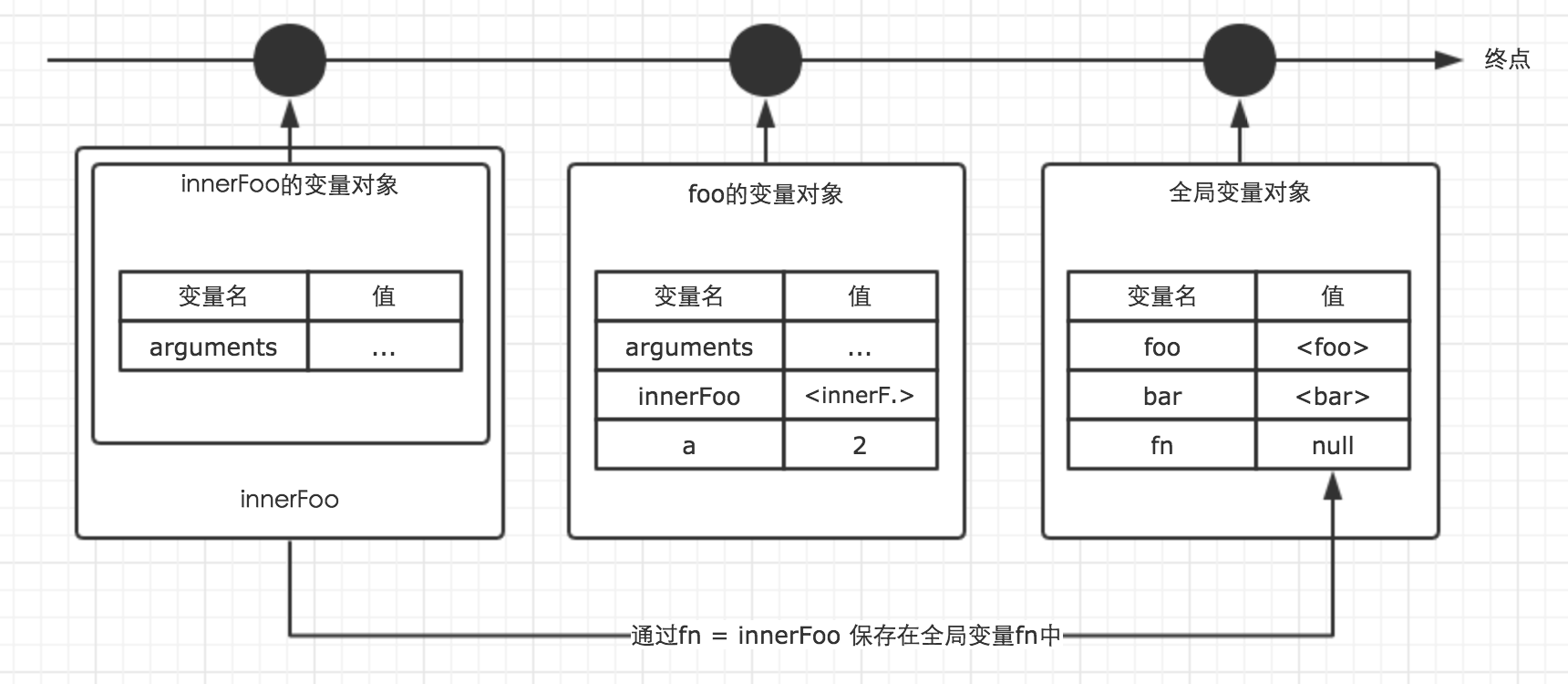# 理解“闭包”

GitHub：zhe-si (哲思) (github.com)

## 离散数学——“闭包”

• 集合：有限、无序、互异的元素组成的整体
• 运算：n个集合映射到某一集合的映射过程
• 关系：多个集合笛卡儿积的子集，如三元关系类似 <a, b, c>，可以想象为一个n维矩阵

【维基百科】

• 例如，实数在减法下闭合，但自然数不行（自然数 3 和 7 的减法 3 − 7 的结果不是自然数）。

• 例如，实数是减法运算下的闭合集合，即实数是减法运算下的闭包。而自然数则不是减法下的闭包。

## 函数式编程——“闭包”

### 概念理解

$( define (f\ X)(Y))$

• 参数：X可为任意个，写作：$$x1\ x2\ ……$$

• 返回值：Y可为表达式（如：$$(+\ x\ y)$$，表示$$x + y$$）或函数（如：$$(lambda (x\ y)(+\ x\ y))$$，表示一个接受参数$$x$$$$y$$并返回$$x + y$$的函数）

$(define\ (f\ x\ y)\ (+\ x\ y))$

$(f\ 5\ 1);\ Value:\ 6$

$(define\ (f\ y)\ (lambda\ (x)\ (+\ x\ y)))$

$(f\ 1);\ Value:\ (lambda\ (x)\ (+\ x\ 1))$

$((f\ 1)\ 5);\ Value:\ 6$

【MDN】

【犀牛书】

【维基百科】

### 画龙点睛

1. 函数式编程，允许运行时定义函数

在上面，我们一直没有关注一个事情——我们不是静态的在编译前定义的函数，而是在运行时动态定义的。这是函数式编程的关键之一，也是闭包形成的基础。

在上面lambda演算的例子中，我们每次在某个环境下动态定义新的函数，并将环境保存，进而形成了闭包。

2. 函数的每次调用都会产生一个新的环境

环境产生的时机是每次调用，其局部环境在调用后产生并作为运行时新动态定义的函数的环境。

这里强调一个点——“每次调用”。也就是说，如果只有一次函数调用，在其内定义多个新函数，每个新函数共享环境。

这里是一个很容易出错的地方，来个例题：利用闭包，修改下面的代码，让循环输出的结果依次为1， 2， 3， 4， 5。

该例中，我们期望每一个timer引用的i的取值作为一个独立的环境，这样timer闭包就可以输出不同的数字。

而在下面给定的源代码中，定义的多个新函数绑定的是同一个环境（最外层函数的 i 局部变量），导致每个输出都为i的最终值6。

for (var i = 1; i <= 5; i++) {
setTimeout(function timer() {
console.log(i);
}, i * 1000);
}


我们为每一个 i 的取值添加了一个函数的调用，对应产生了一个独立的环境，每一个新定义的timer都使用独立环境的 ii 变量，实现了我们的目标效果。

for (var i = 1; i <= 5; i++) {
(function () {
var ii = i;
setTimeout(function timer() {
console.log(ii);
}, i * 1000);
})()
}

3. 调用定义新函数的函数才会产生新的闭包

闭包的产生对应一个新的函数被定义出来，与闭包函数本身被调用无关。

这里也有一个例子：

var fn = null;
function foo() {
var a = 2;
function innnerFoo() {
console.log(c); // 在这里，试图访问函数bar中的c变量，会抛出错误
console.log(a);
}
fn = innnerFoo; // 将 innnerFoo的引用，赋值给全局变量中的fn
}

function bar() {
var c = 100;
fn(); // 此处的保留的innerFoo的引用
}

foo();
bar();


本例中，试图在bar函数中调用某闭包函数访问bar内的局部变量，期望让闭包函数本身的调用影响闭包的产生，显然是错误的。

还是那句话，只有调用定义新函数的函数（或者像本例将新定义的函数通过保存在一个变量而不是作为返回值返回）的时候产生的环境才可以被闭包函数引用并产生新的闭包。

4. 一般，闭包绑定的环境只包含用到的部分

也就是说，一般不会不考虑用不用都把环境完整保存（这样没有引用所在外部作用域的非闭包函数岂不也要像闭包一样将环境保存？），而是用了哪个变量就保存那个变量。

举个例子：

我们定义一个两层嵌套的闭包函数c，来探究部分绑定的问题。

python版本

def a():
a1 = 4
a2 = 5

def b():
b1 = 7
b2 = 8

def c():
c1 = 12
c2 = 13
return c1 + b1
# return c1 + a1
return c
return b


js版本

function a() {
var a1 = 1;
var a2 = 2;
return function b() {
var b1 = 11;
var b2 = 12;
return function c() {
var c1 = 101;
var c2 = 102;
return c1 + b1;
// return c1 + a1;
}
}
}


我们只看最内层的闭包函数c。如果引用b1，则返回的函数只包含环境中的b1；如果引用a1，则返回的函数只包含环境中的a1。引用b2、a2同理，大家可以亲手尝试一下。

结果用js进行展示，如下图：### 实现剖析### 类似技术对照

• 函数对象：

c++通过自定义类重载"()"运算符可以实现一种类似函数的调用方式，同时可以自由定义和配置不同对象（修改其内属性的值）来实现"()"运算符调用的不同效果，类似闭包绑定不同环境。

python也可以通过定义“_call_”魔术方法实现类似效果。

• 内部类：

java支持在函数中定义一个内部类，在内部类中可以引用外部函数的局部变量以及外部类的属性。

这个特性，也被java用来支持函数式编程的实现，如java中的lambda表达式。

## 【拓展】数据库——“闭包”

$子集A的闭包 = 子集A \ ∪ \ 子集A通过FD集推导出的所有属性集合$

FD集：$$\{ A \rightarrow B, B \rightarrow C, D \rightarrow B \}$$

• $$A^+ = A B C$$

解释：A可以推导出B，B推导出C，加起来为ABC

• $$(AD)^+ = ABCD$$

解释：A可以推导出B，B推导出C，D推导出B，加起来为ABCD

• $$(BD)^+ = BCD$$

解释：B可以推导出C，D可以推导出B，加起来为BCD

## 后记

posted @ 2022-03-12 23:33  _哲思  阅读(623)  评论(0编辑  收藏  举报